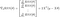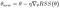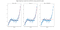# Gradient Descent for Linear Regression from Scratch

## Numpy implementation of Batch Gradient Descent

Cost Function

Our cost function is the residual sum of squares (RSS). If you like it can be modified slightly to be the Mean Squared Errors (MSE), by dividing by the number of instances in the set (since this is a constant, it will not change the calculations below). As a reminder, with feature matrix X, and target vector y, that equation can be written like this:Our Cost Function Residual Sum of Squares (RSS), Image by Author

We start by first choosing a random vector of coefficients theta, and taking the partial derivative of our cost function (often denoted by “J”, we retain RSS here for clarity), with respect to each value of theta. The resulting vector is called the “gradient vector,” since it contains the value of the slopes, or “gradients” measured in each dimension. Remembering our chain rule, we can write that equation like this:Gradient Vector Equation (Image by Author)

At each “step,” we will update our initially random estimate of the values of the coefficient vector, by subtracting the newly computed gradient vector, multiplied by a scalar to control our step size. The scalar for our step size (how far we move in the new direction) is our first hyperparameter and is commonly denoted as “eta.” This updating pattern on each step can be written as follows:The equation for our new coefficient vector theta (Image by Author)

There is another important hyperparameter which controls our stopping criterion. This can be done by measuring whether or not we have already “converged” on a solution (i.e. our new coefficient vector changes less and less until eventually it no longer continues to change very much at all), or as we’ll implement it, by providing the number of iterations we’d like to go through. The code for our batch solution therefore looks like this:Placeholder Alphas (Image by Author)

Data Scientist, lifelong learner, background in Housing Finance, Transportation and Infrastructure.

## More from Jake Miller Brooks

Data Scientist, lifelong learner, background in Housing Finance, Transportation and Infrastructure.